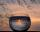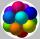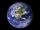# Equilateral cylinder

A sphere is inserted into the rotating equilateral cylinder (touching the bases and the shell). Prove that the cylinder has both a volume and a surface half larger than an inscribed sphere.

Result

k1 =  1.5
k2 =  1.5

#### Solution:Leave us a comment of example and its solution (i.e. if it is still somewhat unclear...):

Showing 0 comments:Be the first to comment!#### To solve this example are needed these knowledge from mathematics:

Tip: Our volume units converter will help you with converion of volume units.

## Next similar examples:

1. Water wellDrilled well has a depth 20 meters and 0.1 meters radius. How many liters of water can fit into the well?
2. Total displacementCalculate total displacement of the 4-cylinder engine with the diameter of the piston bore B = 6.6 cm and stroke S=2.4 cm of the piston. Help: the crankshaft makes one revolution while the piston moves from the top of the cylinder to the bottom and back.
3. Cylinder surface areaVolume of a cylinder whose height is equal to the radius of the base is 678.5 dm3. Calculate its surface area.
4. KitchenKitchen roller has a diameter 70 mm and width of 359 mm. How many square millimeters roll on one turn?
5. PoolThe pool has the shape of a cylinder with a diameter 4.6m, the presumed height is 130cm. The bucket fits 12l of water. How many buckets do you have to bring to fill this pool?
6. RainHow many mm of water rained the roof space 75 m2 if the empty barrel with a radius of 8 dm and height 1.2 m filled to 75% its capacity? :-)
7. Garden pondConcrete garden pond has bottom shape of a semicircle with a diameter 1.7 m and is 79 cm deep. Daddy wants make it surface. How many liters of water is in pond if watel level is 28 cm?
8. Cylinder - A&VThe cylinder has a volume 1287. The base has a radius 10. What is the area of surface of the cylinder?
9. Rotary cylinder 2Base circumference of the rotary cylinder has same length as its height. What is the surface area of cylinder if its volume is 250 dm3?
10. Spheres in sphereHow many spheres with a radius of 15 cm can fits into the larger sphere with a radius of 150 cm?
11. Fit ballWhat is the size of the surface of Gymball (FIT - ball) with a diameter of 65 cm?
12. GasholderThe gasholder has spherical shape with a diameter 20 m. How many m3 can hold in?
13. Sphere fallHow many percent fall volume of sphere if diameter fall 10×?
14. Earth's surfaceThe greater part of the earth's surface (r = 6371 km) is covered by oceans; their area is approximately 71% of the Earth's surface. What is the approximate area of the land?
15. Sphere growthHow many times grow volume of sphere if diameter rises 10×?
16. Cone area and sideCalculate the surface area and volume of a rotating cone with a height of 1.25 dm and 17,8dm side.
17. Cube volumeThe cube has a surface of 384 cm2. Calculate its volume.